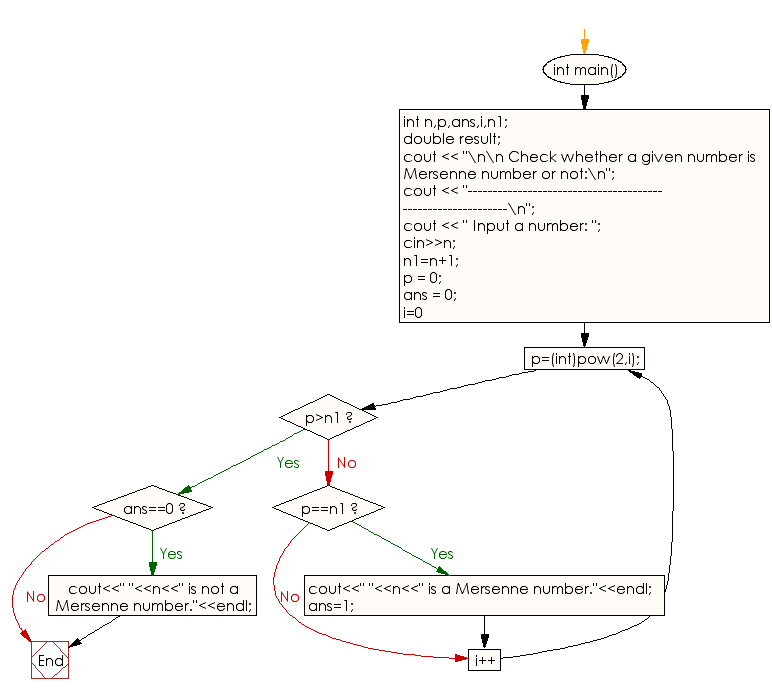﻿ C++ Exercises: Check if a number is Mersenne number or not - w3resource

# C++ Exercises: Check if a number is Mersenne number or not

## C++ Numbers: Exercise-35 with Solution

Write a C++ program to check if a number is a Mersenne number or not.

Sample Solution:

C++ Code :

``````# include <iostream>
# include <math.h>
using namespace std;
int main()
{
int n,p,ans,i,n1;
double result;
cout << "\n\n Check whether a given number is Mersenne number or not:\n";
cout << "------------------------------------------------------------\n";
cout << " Input a number: ";
cin>>n;
n1=n+1;
p = 0;
ans = 0;
for(i=0;;i++)
{
p=(int)pow(2,i);
if(p>n1)
{
break;
}
else if(p==n1)
{
cout<<" "<<n<<" is a Mersenne number."<<endl;
ans=1;
}
}
if(ans==0)
{
cout<<" "<<n<<" is not a Mersenne number."<<endl;
}
}
``````

Sample Output:

``` Check whether a given number is Mersenne number or not:
------------------------------------------------------------
Input a number: 31
31 is a Mersenne number.
```

Flowchart:C++ Code Editor:

What is the difficulty level of this exercise?

﻿

## C++ Programming: Tips of the Day

How do I iterate over the words of a string?

For what it's worth, here's another way to extract tokens from an input string, relying only on standard library facilities. It's an example of the power and elegance behind the design of the STL.

```#include <iostream>
#include <string>
#include <sstream>
#include <algorithm>
#include <iterator>

int main() {
using namespace std;
string sentence = "And I feel fine...";
istringstream iss(sentence);
copy(istream_iterator<string>(iss),
istream_iterator<string>(),
ostream_iterator<string>(cout, "\n"));
}
Instead of copying the extracted tokens to an output stream, one could insert them into a container, using the same generic copy algorithm.

vector<string> tokens;
copy(istream_iterator<string>(iss),
istream_iterator<string>(),
back_inserter(tokens));
... or create the vector directly:

vector<string> tokens{istream_iterator<string>{iss},
istream_iterator<string>{}}; ```

Ref : https://bit.ly/3un4O6f

We are closing our Disqus commenting system for some maintenanace issues. You may write to us at reach[at]yahoo[dot]com or visit us at Facebook